International Journal of Materials Science and Applications
Volume 4, Issue 5, September 2015, Pages: 360-363

Equation Chapter 1 Section 1 Distillation of Two-Photon Entanglement by Anisotropic Metamaterial

Yunxia Dong

School of Electrical and Electronic Engineering, North China Electric Power University, Beijing, China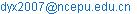Yunxia Dong. Equation Chapter 1 Section 1 Distillation of Two-Photon Entanglement by Anisotropic Metamaterial.International Journal of Materials Science and Applications. Vol.4, No. 5, 2015, pp. 360-363. doi: 10.11648/j.ijmsa.20150405.22

Abstract: By using the Green-function approach to the quantization of the electromagnetic field, the procrustean distillation for quantum entanglement by the anisotropic magnetodielectric metamaterial has been proposed. We study the distillation of the non-maximally entangled pure states by a single layer of the anisotropic magnetodielectric metamaterial. The Clauser–Horne–Shimony–Holt parameter S for the entangled pure state has been calculated theoretically. It is shown that near the resonant peak of transmission for the y-polarized photon, the parameter S for the output states get the maximal value. Near the resonant peak of the transmission for the x-polarized photon, the entanglement of the output state is lower than the input state. For the frequency far from the resonant peak, the entanglement of the output state is dependent on the input state. Finally we find that the maximally entangled output is always appearing near the resonant frequency of the transmission for the y-polarized photon, no matter how low the entanglement of the input state is. This means that our method to realize the quantum distillation is effective.

Keywords: Anisotropic Metamaterial, Magnetodielectric, Quantization, Entanglement Distillation

Contents

1. Introduction

In recent years different quantization approaches such as the damped polarization model  and the phenomenological method  for the electromagnetic field in absorptive and dispersive dielectrics have been developed. The damped polarization model to quantize the electromagnetic field is a canonical quantization in which the medium is represented by a collection of interacting matter fields and the absorptive character of the medium is described by the interaction of the matter fields with a heat bath containing a continuum of reservoir fields . The phenomenological scheme has been formulated on the basis of the fluctuation-dissipation theorem. The combination of the Maxwell equations and the constitutive equation in the frequency domain gives the electromagnetic field operators in terms of the noise polarization field and the classical Green tensor .

Recently, the metamaterials have attracted a great deal of attention from both theoretical and experimental sides. The metamaterials have the artificial structure and the electromagnetic parameters are dependent on the resonant of the electric and the magnetic field. These media exhibit a number of unusual electromagnetic properties such as negative refractive index  amplification of evanescent wave , subwavelength cavity resonator , zero averaged refractive index band gap  etc. Due to that the unit resonance structures of metamaterials are usually anisotropic , people became interested in the anisotropic properties and revealed many intriguing phenomena in different kinds of anisotropic metamaterials [7-11]. Based on phenomenological method, the quantization of the electromagnetic field in anisotropic magnetodielectric metamaterials has been performed .

In this work we investigate the propagation of quantized radiation through anisotropic magnetodielectric metamaterial and explore the possibility to control the polarization entangled photon pairs or realize the distillation of the entanglement. In fact, various schemes of entanglement distillation, purification and concentration have been proposed [13-20]. Some of the schemes have been realized by experiments and the most successful example is the procrustean distillation of the entangled pure state by using partial polarizer [16,17]. However, our investigations show that it is effective to realize the quantum distillation by the anisotropic magnetodielectric metamaterial.

2. Theory of Distillation by Anisotropic Metamaterial

We consider a single slab of anisotropic magnetodielectric metamaterial with the permittivity tensor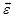and permeability tensor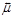embedded in the dielectric background (air). The interface of the metamaterial plate is parallel to the x-y plane. Introducing the vector potential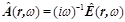, from the Maxwell equations we get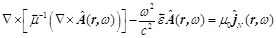(1)

The solution of Eq.(1) can be given by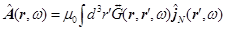(2)

where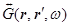is the Green tensor satisfying the equation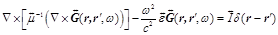(3)

Here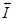is the three order unit tensor.

Assuming the photon travels along the z-axis, the relations between outgoing fields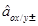and incoming fields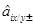are determined by definitions of the operators with the boundary continuity conditions of the vector potential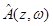at the interface. The input-output relations for the amplitude operators through the single-layer structure can be obtained as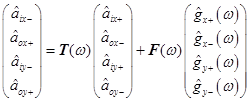(4)

Here, we consider that the incident two-photon state has a general form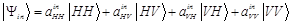(5)

where H and V represent horizontal and vertical polarization, respectively. The complex coefficients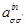(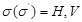) form a matrix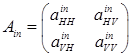(6)

The relation between the output state and the input state can be obtained by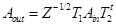(7)

with normalized factor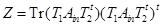(8)

Here superscript "t" denotes the transpose of a matrix, and the transmission matrix is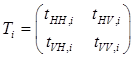(9)

where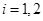denotes different channels.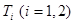can be get from the Eq. (4).

After the transmission coefficients are obtained, the degrees of the entanglement for input and output pure states can be obtained in terms of the Clauser-Horne-Shimony-Holt (CHSH) parameter S. According to Ref. , the parameter S for the input and output states can be expressed as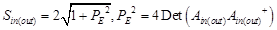(10)

with the superscript "+" being the transposed and complex conjugate matrix and "Det" being the determinant.

A maximally entangled state has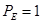,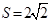, and the disentangled state has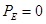,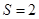. So if input state with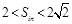is distilled to a state with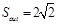, this means that we have distilled the non-maximally entangled state to the maximally entangled state successfully. For example, a two-qubit state of the form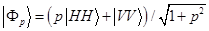(11)

which is not maximally entangled state if p is not equal to 1, can be transformed into the maximally entangled state with a certain probability by tuning the parameters of the anisotropic metamaterial. The distillation does not increase entanglement, but concentrates the entanglement into a smaller number of maximally entangled states. It is necessary to sacrifice some photon pairs to obtain the more entangled states. The probability for the initial photon pairs surviving the filtering process can be described by the normalized factor which depends on the input state and the transmission of the filtering devices. In the ideal situation, the distillation devices have no effect on the horizontal polarized photon and transmit the vertical polarized photon with probability. Thus the probability for the yield of the output maximally entangled pair is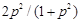. With the increase of p, the survival probability increases monotonically. This means that the more entanglement the input state has, the larger the survival probability is. We will present the concrete calculated results in the next part.

3. Results and Discussions

In the following, we present the calculated results for above theory. First, we consider the distillation of the non-maximally entangled pure states by the anisotropic metamaterial. We can produce entangled photon pairs by pumping a 351 nm laser beam on two adjacent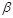-barium borate (BBO) crystals in a state of Eq. (11). By simple varying the pump polarization, the value of p can be set arbitrarily. Here we choose the coordinates that H corresponds to the polarization along the x-axis, and V to that along the y-axis. Let us consider that the non-maximally entangled pure state is incident on a single layer of anisotropic metamaterial with the permeability and dielectric tensors given by Ref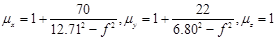(12)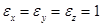(13)

where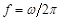is the frequency measured in gigahertz.

The calculated results for the transmissions of different polarized waves as a function of the frequency are plotted in Fig.1 (a). The thickness of the metamaterial is taken as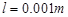. There is a resonant peak of the transmission appearing near the resonant frequency. For the x- (y-) polarized wave, the resonant peak of the transmissions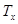(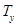) exhibits at the resonant frequency of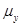(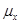). The CHSH parameter S for the output states with p=0.5 is plotted in Fig.1 (b). Near the resonant peak of the transmission for the y-polarized photon, the CHSH parameter S for the output state gets the maximal value.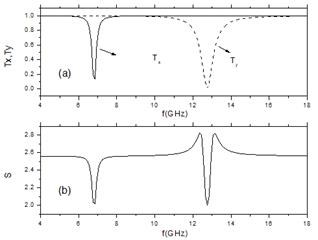Fig. 1. The transmissions of different polarized waves as a function of the frequency are plotted in Fig.1 (a); The CHSH parameter S for the output states with p=0.5 is plotted in Fig.1 (b).

Figure 2 describes the CHSH parameter S as a function of the frequency for different p. The thickness of the metamaterial is taken also as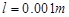. Fig.2 (a), (b) and (c) correspond to the input state with p=0.2 (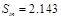), 0.5 (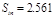) and 0.8 (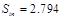), respectively. From the results we find that for the frequency far from the resonant peak, the entanglement of the output state is dependent on the input state. Near the resonant peak of the transmission for the x-polarized photon, the entanglement of the output state is lower than the input state. The entanglement of the output state shows the resonant peak near the resonant peak of the transmission for the y-polarized photon. This is because the input state is in form of Eq. (11) and the component for the y-polarized wave is more than the x-polarized wave.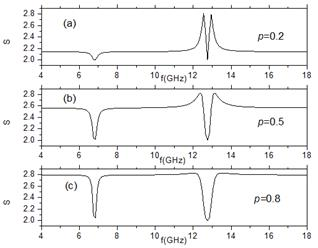Fig. 2. The corresponding results as a function of the frequency for different p with the thickness of the metamaterial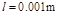.

In order to get the relation between the distillation and the thickness of the metamaterial, we plot the CHSH parameter S as a function of the frequency in Fig. 3 for the input states with p=0.5 for three cases with different thickness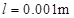(a),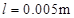(b) and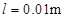(c). The results show that the maximally entangled output is always appearing near the resonant frequency of the transmission for the y-polarized photon, no matter how low the entanglement of the input state is.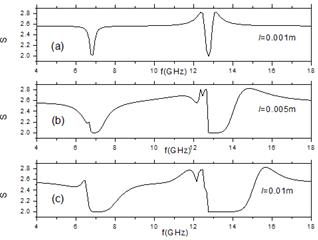Fig. 3. The corresponding results as a function of the frequency for different thickness of the metamaterial with p=0.5.

4. Conclusion

By using the Green-function approach to the problem of quantization of the electromagnetic field, the effective method to perform procrustean distillation for quantum entanglement by the anisotropic magnetodielectric metamaterial has been proposed. We study the distillation of the non-maximally entangled pure states by a single layer of the metamaterial. The Clauser–Horne–Shimony–Holt parameter S for an entangled pure state has been calculated theoretically. It is shown that near the resonant peak of transmission for the y-polarized photon, the CHSH parameter S for the output states get the maximal value. Near the resonant peak of the transmission for the x-polarized photon, the entanglement of the output state is lower than the input state. For the frequency far from the resonant peak, the entanglement of the output state is dependent on the input state. The maximally entangled output is always appearing near the resonant frequency of the transmission for the y-polarized photon, no matter how low the entanglement of the input state is. Thus our method to use the metamaterial to the distillation of the entangled state is an effective way.

Acknowledgements

Project supported by the National Natural Science Foundation of China (Grant No. 11104071) and the Fundamental Research Funds for the Central Universities (Grant No. 2015MS01).

References

1. B. Huttner and S. M. Barnett, "Quantization of the electromagnetic field in dielectrics," Phys. Rev. A 46, 4306(1992).
2. T. Gruner and D. G. Welsch, "Correlation of radiation-field ground-state fluctuations in a dispersive and lossy dielectric," Phys. Rev. A 51, 3246 (1995).
3. D. R. Smith, W. J. Padilla, D. C. Vier, S. C. Nemat-Nasser, and S. Schultz, "Compositemedium withsimultaneouslynegativepermeability andpermittivity," Phys. Rev. Lett. 84, 4184 (2000).
4. J. B. Pendry, "Negativerefractionmakes aperfectlens," Phys. Rev. Lett. 85, 3966(2000).
5. L. F. Shen, S. L. He, and S. S. Xiao, "Stability and quality factor of a one-dimensional subwavelength cavity resonator containing a left-handed material", Phys. Rev. B 69, 115111(2004).
6. J. Li, L. Zhou, C.T. Chan, P. Sheng, "Photonicbandgap from astack ofpositive andnegativeindexmaterials," Phys. Rev. Lett. 90, 083901 (2003).
7. D. R. Smith and D. Schurig, "Electromagneticwavepropagation inmedia withindefinitepermittivity andpermeabilitytensors," Phys. Rev. Lett. 90, 077405 (2003).
8. L. Zhou, C. T. Chan, and P. Sheng, "Anisotropy and oblique total transmission at a planar negative-index interface," Phys. Rev. B 68, 115424 (2003).
9. S. Sun, X. Huang, and L. Zhou, "Two-dimensional complete photonic gaps from layered periodic structures containing anisotropic left-handed metamaterials," Phys. Rev. E 75,066602 (2007).
10. L. Hu and S. T. Chui, "Characteristics of electromagnetic wave propagation in uniaxially anisotropic left-handed materials," Phys. Rev. B 66, 085108 (2002).
11. J.Hao, Y.Yuan,L.Ran, T.Jiang, J.A.Kong, C.T.Chan and L.Zhou, "Manipulatingelectromagneticwavepolarizations byanisotropicmetamaterials," Phys. Rev. Lett. 99, 063908 (2007).
12. Y.Dong, C.Liu, "Electromagnetic field quantization and input-output relation for anisotropic magnetodielectric metamaterial," Chin. Phys. B 24, 064206(2015).
13. J. L. van Velsen, J. Tworzydlo, and C.W. J. Beenakker, "Scattering theory of plasmon-assisted entanglement transfer and distillation", Phys. Rev. A 68, 043807 (2003).
14. C.H.Bennett, H.J.Bernstein, S.Popescu and B.Schumacher, "Concentrating partial entanglement by local operations," Phys. Rev. A 53, 2046 (1996).
15. J.-W.Pan,C.Simon, et al, "Entanglement purification for quantum communication" Nature 410, 1067 (2001).
16. P.G.Kwiat, S.Barraza-Lopez, A.Stefanov and N.Gisin, "Experimental entanglement distillation and ‘hidden’ non-locality," Nature 409, 1014 (2001).
17. N.A.Peters, J.B.Altepeter, et al, "Maximallyentangledmixedstates:creation andconcentration," Phys. Rev. Lett. 92, 133601 (2004).
18. Y.S.Huang, H.B.Xing, et al, "Distillation of multipartite entanglement by local filtering," Phys. Rev. A 89, 062320 (2014).
19. H.J.Liu, L.L.Fan, et al, "Efficient entanglement concentration for partiallyentangled cluster states with weak cross-Kerr nonlinearity," Quantun Inf. Process 14, 2909 (2015).
20. M.A.al Farooqui,J.Breeland, et al, "Quantum entanglement distillation with metamaterials,"Opt. Express 23, 17941 (2015).

 Contents 1. 2. 3. 4.
Article ToolsAbstractPDF(209K)